# The GLMPOWER Procedure

#### Contrasts in Fixed-Effect Univariate Models

The univariate linear model has the form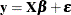where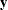is the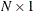vector of responses,is the
design matrix,is the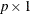vector of model parameters corresponding to the columns of, andis anvector of errors with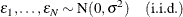In PROC GLMPOWER, the model parametersare not specified directly, but rather indirectly as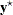, which represents either conjectured response means or typical response values for each design profile. Thevalues are manifested as the dependent variable in the MODEL statement. The vectoris obtained fromaccording to the least squares equation,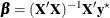Note that, in general, there is not a 1-to-1 mapping betweenand. Many different scenarios formight lead to the same. If you specifywith the intention of representing cell means, keep in mind that PROC GLMPOWER allows scenarios that are not valid cell means according to the model specified in the MODEL statement. For example, ifexhibits an interaction effect but the corresponding interaction term is left out of the model, then the cell means (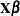) derived fromdiffer from. In particular, the cell means thus derived are the projection ofonto the model space.

It is convenient in power analysis to parameterize the design matrixin three parts,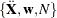, defined as follows:

1. The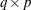essence design matrix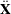is the collection of unique rows of. Its rows are sometimes referred to as design profiles. Here,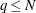is defined simply as the number of unique rows of.

2. The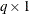weight vectorreveals the relative proportions of design profiles. Row i ofis to be included in the design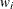times for every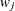times row j is included. The weights are assumed to be standardized (that is, sum up to 1).

3. The total sample size is N. This is the number of rows in. If you gather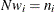copies of the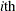row of, for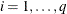, then you end up with.

It is useful to express the crossproduct matrixin terms of these three parts,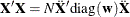since this factors out the portion (N) depending on sample size and the portion (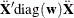) depending only on the design structure.

A general linear hypothesis for the univariate model has the form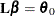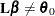whereis an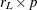contrast matrix (assumed to be full rank) and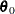is the null value (usually just a vector of zeros). Note that effect tests are just contrasts that use special forms of. Thus, this scheme covers both effect tests and custom contrasts.

The test statistic is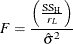where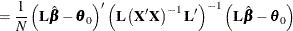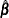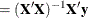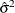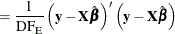where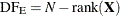. Note that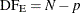ifhas full rank.

Under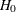,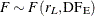. Under
, F is distributed as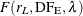with noncentrality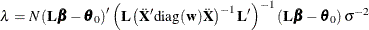Muller and Peterson (1984) give the exact power of the test as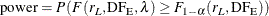Sample size is computed by inverting the power equation.

See Muller and Benignus (1992) and O’Brien and Shieh (1992) for additional discussion.